Examples for Resistors

Example #1

Problem:

A wire of length L = 60 m needs to be laid to carry a current of 150 amps with a voltage drop of no more than 0.5 V. The wire is to be made of copper which has a resistivity r = 1.72E-8 Wm. What is the minimum diameter D of the wire?

Solution:

The resistance of the wire needs to by less than the voltage drop divided by the current.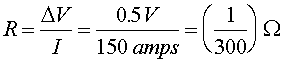The resistance is given by R = rL/A, therefore the cross sectional area is: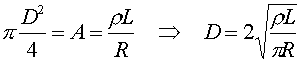D = 1.99 cm

Example #2

Problem:

Consider the circuit below. What is the equivalent resistance Rab?DATA: R1 = R2 = R3 = 7.0 W

Solution:

First consider the upper two resistors as a single resistor, R23. The resistance RAB can be found by adding R1 and R23 in parallel.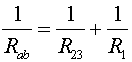Adding one 1/14 and 1/7 gives 3/14, therefore

RAB = (14/3) W

Example #3

Problem:

What is the equivalent resistance RAB of the three resistors shown below.Data R1 = R2 = R3 = 6 W.

Solution:

Use the relation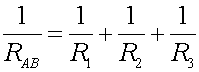to solve for RAB.

RAB = 2 W

Example #4

Problem:

A 12-volt battery is used to heat a 120 ml cup of coffee by submerging an 8.0-ohm resistor. How long will take to bring the coffee from 20 oC to 100 oC?

Solution:

The energy needed to heat the water is: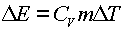where Cv is the specific heat, one calorie per gram, DT is the change in temperature and m is the mass, 120 g. One then obtains the energy, E = 9600 calories = 4.03E4 J. The time Dt can be found by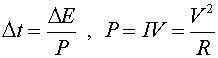Dt = 37 minutes

Resistor's index